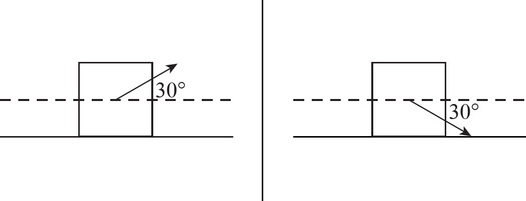# AP Physics 1 Question 322: Answer and Explanation

### Test Information

Question: 32210. Pulling a block of mass m to the right by a connected string at an angle of 30 degrees above the horizontal (as shown in the left picture) with a force equal to the block's weight produces a friction force Fkf. If the same block were to be pulled at an angle of 30 degrees beneath the horizontal (as shown in the right picture), what would be the friction force? Assume that the applied force is enough to make the block move in both cases.

• A. 3Fkf
• B. 2Fkf
• C. Fkf/2
• D. Fkf/3